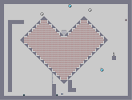The Great HeartHover over the thumbnail for a full-size version.

Author Malavin action author:malavin playable unrated 2006-05-25 4 more votes required for a rating. \$The Great Heart#Malavin#none#00000000000000000000000000011111111111111111100000100000000000000000000001000000000000000000000010003400000000000000000000300400000000000000000030000400000000000000003000000400000000000000300000000400000000000030000000000400000000000200000000000400000000000200000000000400000000000200000000000400111000000200000000000400000000000200000000000400000000003000000000005000000000300000000000511100000030000000000050001000003000000000005000000000300000000000500000000002000000000050000000000002000000005000000000000002000000500000000000000002000050000000000000000002005000000000000000000002500000000000000000000000000000000000000000000000001000000000000000000000000000000000000000000000000000000000000000000000000000000|5^684,324!12^252,132!12^264,132!12^276,132!12^276,144!12^264,144!12^252,144!12^228,156!12^372,468!12^384,468!12^396,468!12^396,456!12^372,456!12^396,456!12^384,456!12^372,444!12^360,444!12^348,444!12^348,432!12^348,420!12^348,408!12^348,396!12^348,384!12^348,372!12^348,360!12^384,228!12^384,240!12^384,252!12^384,264!12^384,276!12^384,288!12^384,300!12^384,312!12^384,324!12^384,336!12^384,348!12^384,360!12^384,372!12^384,384!12^384,396!12^384,408!12^384,420!12^384,432!12^384,444!12^396,444!12^396,432!12^396,420!12^396,396!12^396,228!12^396,240!12^396,252!12^396,264!12^396,264!12^396,288!12^396,264!12^396,288!12^396,276!12^396,300!12^396,312!12^396,324!12^396,336!12^396,348!12^396,360!12^396,372!12^396,384!12^396,408!12^408,444!12^420,444!12^420,420!12^420,432!12^420,408!12^420,384!12^420,396!12^156,240!12^156,228!12^156,252!12^168,252!12^180,264!12^180,276!12^192,276!12^204,276!12^204,288!12^204,300!12^216,300!12^228,312!12^228,300!12^228,324!12^240,156!12^252,156!12^276,156!12^264,156!12^288,156!12^300,156!12^300,168!12^300,180!12^312,180!12^324,180!12^324,192!12^324,204!12^348,204!12^336,204!12^348,216!12^348,228!12^360,228!12^372,228!12^408,228!12^420,216!12^420,288!12^408,336!12^276,264!12^204,204!12^288,288!12^336,360!12^456,336!12^492,312!12^480,276!12^504,252!12^528,240!12^540,216!12^540,216!12^492,216!12^480,264!12^516,252!12^528,252!12^504,288!12^492,312!12^552,288!12^564,240!12^516,192!12^468,192!12^492,180!12^468,276!12^408,324!12^348,300!12^300,288!12^336,336!12^336,360!12^312,372!12^252,300!12^252,276!12^288,240!12^264,252!12^240,216!12^276,228!12^312,252!12^324,276!12^264,264!12^228,228!12^216,240!12^264,276!12^348,276!12^336,324!12^264,300!12^252,228!12^276,216!12^324,240!12^264,240!12^252,228!12^252,228!12^252,228!12^252,240!12^336,264!12^324,312!12^276,324!12^288,336!12^312,372!12^288,372!12^240,300!12^240,276!12^276,264!12^240,264!12^228,240!12^240,240!12^216,240!12^216,240!12^216,240!12^312,288!12^276,288!12^276,288!12^276,288!12^276,288!12^276,288!12^276,288!12^252,252!12^264,252!12^204,204!12^192,216!12^216,252!12^228,252!12^276,288!12^360,312!12^372,300!12^384,228!12^504,228!12^504,228!12^432,288!12^444,228!12^444,228!12^468,252!12^420,288!12^444,276!12^444,240!12^408,252!12^468,264!12^480,300!12^516,300!12^420,336!12^444,324!12^432,360!12^420,360!12^180,204!12^192,204!12^192,192!12^204,192!12^204,180!12^216,180!12^228,168!12^288,336!12^312,312!12^240,168!12^252,168!12^264,168!12^276,168!12^288,168!12^300,192!12^312,192!12^312,204!12^324,216!12^324,228!12^336,216!12^336,240!12^336,228!12^348,240!12^360,240!12^372,240!12^372,252!12^372,264!12^372,276!12^372,288!12^372,300!12^372,312!12^372,324!12^372,336!12^372,348!12^372,360!12^372,372!12^372,384!12^372,396!12^372,408!12^372,420!12^372,432!12^360,432!12^360,420!12^360,408!12^360,396!12^360,384!12^360,372!12^360,348!12^360,360!12^360,336!12^360,324!12^360,300!12^360,288!12^360,276!12^360,264!12^360,252!12^348,252!12^336,252!12^324,252!12^324,264!12^324,288!12^324,300!12^324,336!12^324,324!12^324,360!12^324,348!12^324,372!12^324,396!12^324,384!12^324,408!12^324,420!12^336,420!12^336,408!12^336,408!12^336,396!12^336,384!12^336,372!12^336,348!12^336,312!12^336,300!12^336,288!12^336,276!12^348,264!12^348,288!12^348,312!12^348,324!12^348,336!12^348,348!12^408,432!12^408,408!12^408,384!12^408,240!12^408,264!12^408,276!12^408,288!12^408,300!12^408,312!12^408,348!12^408,372!12^408,360!12^408,396!12^408,420!12^432,420!12^432,432!12^456,408!12^480,384!12^504,360!12^240,144!12^216,168!12^168,216!12^144,240!12^144,252!12^144,228!12^168,264!12^192,288!12^216,312!12^240,336!12^264,360!12^288,384!12^312,408!12^336,432!12^360,456!12^384,480!12^396,480!12^372,480!12^408,456!12^528,336!12^552,312!12^576,288!12^600,264!12^624,240!12^600,216!12^576,192!12^552,168!12^528,144!12^492,120!12^504,120!12^516,120!12^480,144!12^456,168!12^432,192!12^408,216!12^360,216!12^336,192!12^312,168!12^288,144!12^252,120!12^264,120!12^276,120!12^168,228!12^168,240!12^180,252!12^180,240!12^180,228!12^180,216!12^192,228!12^192,240!12^192,252!12^192,264!12^204,264!12^204,252!12^204,240!12^204,228!12^204,216!12^216,192!12^216,216!12^216,204!12^216,240!12^216,228!12^216,264!12^216,276!12^216,288!12^240,324!12^240,288!12^228,288!12^228,276!12^228,264!12^228,216!12^228,204!12^228,192!12^228,180!12^240,180!12^240,192!12^240,204!12^240,228!12^240,252!12^252,348!12^276,372!12^300,396!12^444,420!12^468,396!12^492,372!12^516,348!12^540,324!12^564,300!12^588,276!12^612,252!12^612,228!12^588,204!12^564,180!12^540,156!12^516,132!12^504,132!12^492,132!12^444,180!12^420,204!12^240,312!12^252,180!12^252,192!12^252,216!12^252,204!12^252,264!12^252,288!12^252,312!12^252,336!12^252,324!12^264,348!12^264,336!12^264,312!12^264,324!12^264,288!12^264,228!12^264,204!12^264,180!12^264,192!12^264,216!12^276,180!12^276,192!12^276,204!12^276,240!12^276,252!12^276,276!12^276,312!12^276,300!12^276,336!12^276,348!12^276,360!12^288,360!12^288,324!12^288,348!12^288,312!12^288,276!12^288,300!12^288,264!12^288,252!12^288,228!12^288,204!12^288,192!12^288,180!12^300,384!12^300,372!12^300,360!12^300,348!12^300,324!12^300,216!12^288,216!12^300,204!12^300,228!12^312,216!12^300,228!12^300,240!12^312,228!12^300,252!12^300,252!12^300,252!12^300,252!12^300,252!12^300,252!12^300,252!12^300,252!12^300,264!12^312,264!12^312,276!12^300,276!12^300,300!12^312,300!12^300,312!12^312,324!12^300,336!12^312,336!12^312,360!12^312,348!12^312,396!12^312,384!12^432,408!12^432,396!12^432,384!12^432,372!12^432,348!12^432,336!12^432,324!12^432,312!12^432,300!12^432,276!12^432,264!12^432,252!12^432,240!12^432,216!12^432,228!12^432,204!12^444,192!12^456,180!12^468,168!12^480,156!12^492,144!12^504,144!12^516,144!12^528,156!12^540,168!12^552,180!12^564,192!12^576,204!12^588,216!12^600,228!12^612,240!12^600,252!12^588,264!12^576,276!12^444,408!12^456,396!12^468,384!12^480,372!12^492,360!12^504,348!12^504,336!12^516,336!12^528,324!12^540,312!12^552,300!12^564,288!12^444,396!12^420,372!12^420,348!12^420,324!12^420,312!12^420,300!12^420,276!12^420,264!12^420,252!12^420,240!12^420,228!12^312,240!12^492,156!12^492,168!12^492,192!12^492,204!12^492,228!12^492,240!12^492,252!12^492,264!12^492,276!12^492,288!12^492,300!12^492,324!12^492,348!12^492,336!12^444,384!12^444,372!12^444,360!12^444,336!12^444,348!12^444,312!12^444,288!12^444,300!12^444,264!12^444,252!12^444,216!12^444,204!12^480,168!12^480,180!12^468,156!12^480,192!12^480,216!12^480,204!12^480,228!12^480,252!12^480,240!12^480,288!12^480,300!12^480,312!12^480,324!12^480,336!12^480,348!12^480,360!12^540,276!12^540,228!12^540,192!12^552,216!12^528,192!12^528,180!12^516,168!12^516,156!12^504,204!12^516,228!12^468,300!12^468,240!12^468,216!12^468,216!12^468,204!12^468,180!12^468,228!12^468,288!12^468,312!12^468,336!12^468,324!12^468,348!12^468,360!12^468,372!12^456,384!12^456,372!12^456,360!12^456,348!12^456,324!12^456,312!12^456,300!12^456,288!12^456,276!12^456,264!12^456,252!12^456,240!12^456,228!12^456,216!12^456,204!12^456,192!12^504,156!12^504,180!12^504,168!12^504,192!12^504,216!12^504,240!12^504,264!12^516,276!12^504,276!12^504,300!12^504,312!12^504,324!12^516,324!12^516,312!12^516,288!12^516,264!12^516,240!12^516,228!12^516,204!12^516,216!12^516,180!12^540,168!12^528,168!12^540,180!12^540,192!12^540,216!12^540,204!12^540,240!12^540,264!12^540,252!12^540,288!12^540,300!12^528,312!12^528,300!12^528,288!12^528,276!12^528,264!12^528,228!12^528,204!12^528,216!12^552,192!12^600,240!12^588,240!12^576,240!12^552,240!12^552,276!12^552,264!12^552,228!12^552,204!12^552,252!12^576,264!12^576,252!12^576,228!12^576,216!12^564,204!12^564,216!12^564,228!12^564,252!12^564,264!12^564,264!12^564,276!12^588,228!12^588,252!11^384,192,372,564!7^324,492,2!7^324,468,2!7^324,444,2!10^756,120!6^252,84,0,0,1,2!4^342,570,1!6^420,36,0,1,0,2!6^612,420,1,1,0,1!4^144,570,1!6^540,60,1,0,1,NaN# My first attempt ever at making a map. Run quickly.